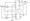# Logic design June 2010

Note: Answer any FIVE full questions, selecting at least TWO questions from each part.

PART-A

1 a. Simplify the following expressions using k – map. Implement the simplified expression

Using basic gates :

i)  T = f(wxyz) = Zm(2,3, 4, 5,13, 15) + £d(8, 9, 10, 11)

ii)   R – f(abcd) = 7iM(2, 3, 4, 6, 7, 10, 11, 12).

b. Place the following equations into proper canonical form :

i) P – f(a, b, c) ~ ab’+ ab’+ be

ii) T = f(a, b, c) (a + b’) (b’ + c).    (04 Marks)

c.  Define the following terms :

i) Minimum iii) Canonical sum of products

ii) Maximum iv) Canonical product of sum.

2 a. Simplify the logic function given below using variable entered mapping (VEM) technique :

f(a,b, c, d) = Zm(2, 9, 10, 11, 13, 14, 15).

b. Simplify the following function using Quine-McClusky minimization technique :

T = f(a, b, c, d) = Xm(0, 1, 2, 3, 6, 7, 8, 9, 14,15).

3 a. Design a combinational logic circuit to output the 2’s compliment of a 4-bit binary numbers:

i)     Construct the truth table

ii)    Simplify each output function using k-map and write reduced equations

iii) Draw the resulting logic diagram.

b.  Construct a scheme to obtain a 4-to-16 line decoder using 74138 (3-8 line decoder).

c. Write a note on encodes.

4 a. Realize the following Boolean function f(ABC) = £(0, 1, 3, 5, 7) using,

i) 8 : lmux(74151)

ii) 4 : lmux(74153).

b.  Design a comparator to check if two n-bit numbers are equal. Configure this using cascaded stage of 1 bit equality comparator.

c. Implement full subtractor using gates and write a truth table.

PART-B

5 a. Explain the operation of SR latch. Explain one of its applications.

b. Draw the logic diagrams for

i) Gated SR latch iii) Master slave JK flip flop

ii) Master slave SR flip flop iv) Positive edge triggered {D’ flip flop.

6 a. Differentiate between combinational logic circuit and sequential logic circuits.

b.  Explain universal shift register with the help of logic diagram, mode control table and symbol.

c.  Explain Jonson counter, with its circuit diagram, and timing diagram.

7 a. Expten Moore and Meclay models for clocked synchronous sequential circuits.

b. Constant the excitation table, transition table, state table and state diagram, for the Moore sequsmtM circuit shown in Fig. Q7(b).Fig. Q 7(b)

8 a. Write a note on construction of state diagram.

b.  Design a counter using JK-flip flops whose counting sequence is 000, 001, 100, 110, 111, 101, 000 etc., by obtaining its minimal sum equations.

c. Write a note on characteristic equations.